Monday, October 2, 2023

# Closed Traverse | Numerical of Closed Traverse | Plotting of Closed Traverse

Simply, Traverse means passing across points.

The series of connected straight lines each joining two points on the ground is called a Traverse.

Endpoints are traverse stations whereas straight lines between two consecutive stations are known as Traverse legs.

Traversing is the type of surveying connecting several survey lines that form a framework

### 1.1. Types of Traverse

There are two types of Traverse. They are explained below in brief.

#### a. Closed Traverse:

If the traverse formed by the survey lines closes at a station i.e. if the survey lines form a polygon or start and finish at the same survey station of the known coordinates then the traverse is known as closed traverse. Closed traversing is generally used for locating the boundaries or for surveying large areas.

#### b. Open Traverse:

If a traverse starts and finishes at points other than the starting point of known coordinates, then such traverse is known as an open traverse. An open traversing is generally used for preliminary and construction surveys for highways, roads, pipelines, transmission lines, etc.

### 1.2. Methods of traversing

Traversing can be done in several methods based on instruments used for surveying to find linear measurement and direction of lines. Some of the methods are as follows:

a. Chain traversing (can be done by using tape or chain)

b. Compass traversing (by using surveyor’s compass)

c. Theodolite traversing

d. Plane-table traversing

### 1.3. Bearing

The horizontal angle between the reference meridian (North or South) and the survey line drawn from the station in a clockwise or anticlockwise direction based on the designation of angles with horizontal is known as a bearing. It is classified into two types i.e. Fore Bearings (F.B.) and Back Bearings (B.B).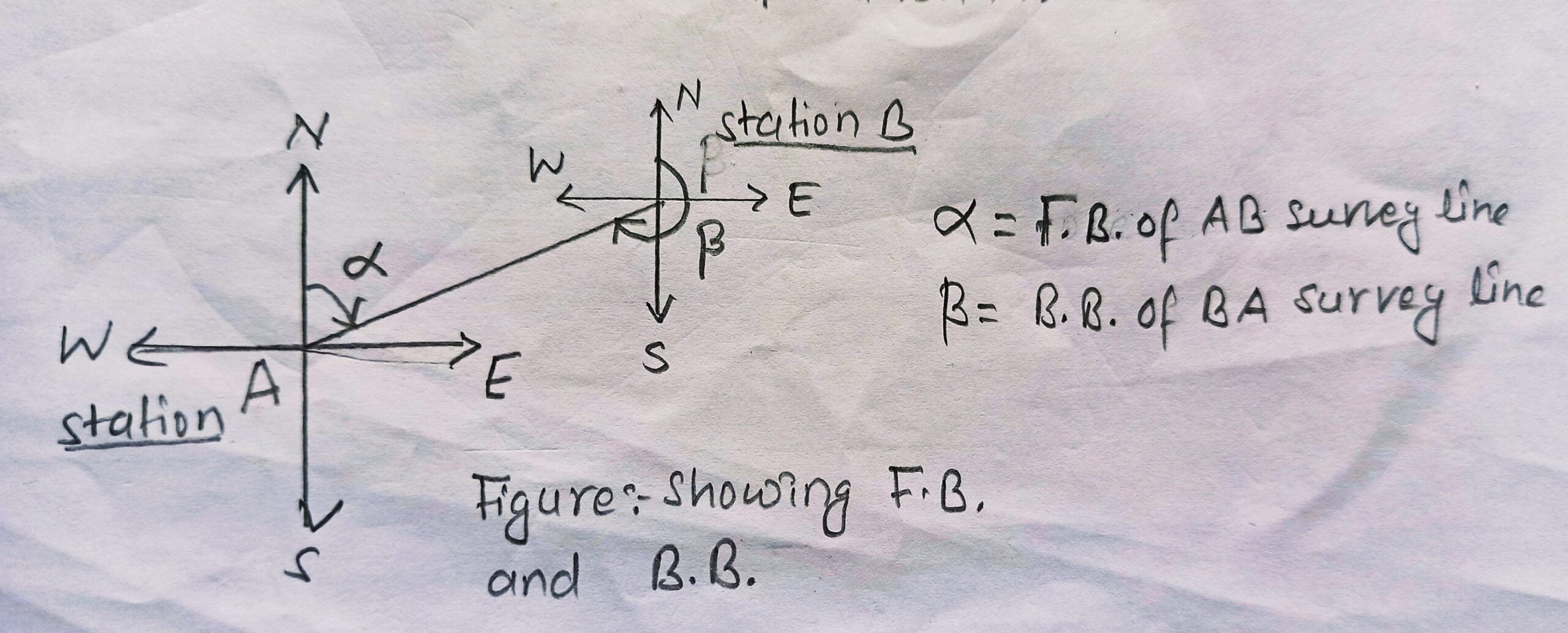In the figure, A and B station; AB and BA are survey lines.

α = Fore bearing of AB

ß = Back bearing of BA

Mathematically,

Back bearing= Fore Bearing ± 180°

Note: Here we use +ve sign if F.B. is less than 180° and -ve  if F. B. is more than 180°.

### 1.4. Meridian

Meridian is the fixed reference direction on the earth to which bearings of survey lines can be expressed. There are four reference meridians used in surveying. They are:

a. True Meridian

b. Magnetic Meridian

c. Grid Meridian

d. Arbitrary Meridian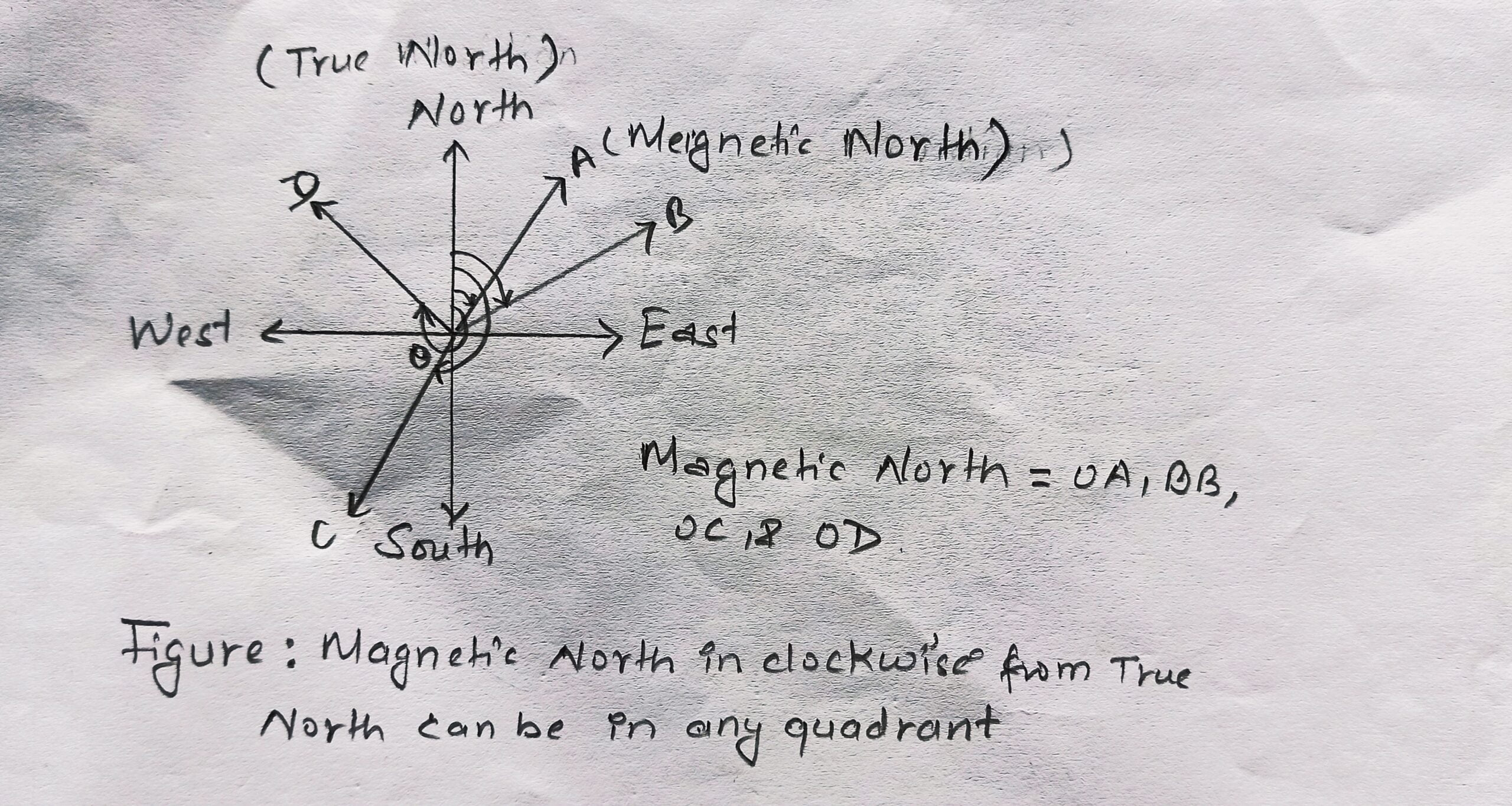Figure: Showing true Meridian & magnetic meridian relation.

### 1.5. Designation of Bearings

Bearings can be designed into two systems. They are as follows:

a. Whole Circle Bearing (WCB)System

In this system, the bearing of the survey line is measured from reference true north (magnetic North of the earth) in a clockwise direction. The value of a bearing may vary from 0° to 360° graduation in WCB.

Prismatic compass is associated with WCB system graduation. This system always starts its bearing from the North direction. Its bearing can be expressed in degree sexagesimal system like degree(°), minute(’) and second(”).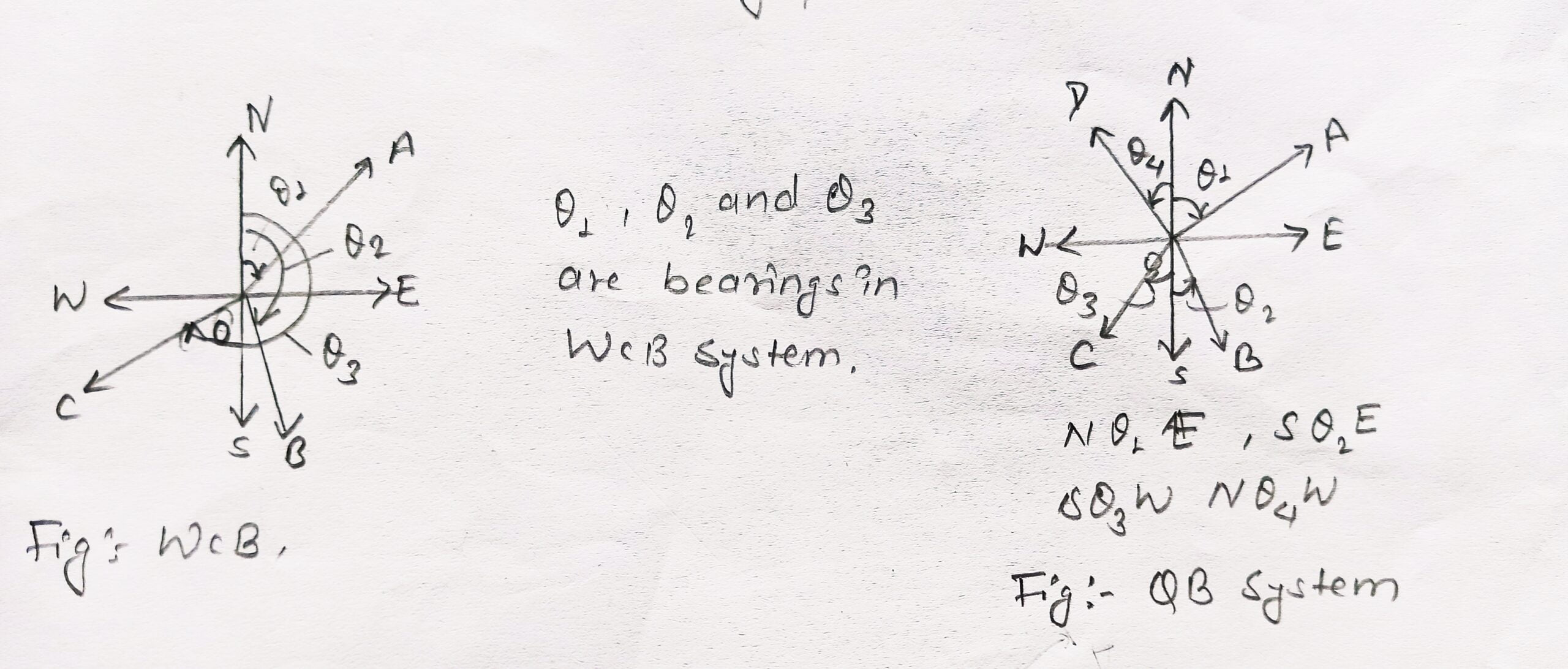b. Quadrantal Bearing (QB) System    (Reduced Bearing, RB)

In this system, the bearing of the survey line is measured from eastward or westward from North or South whichever reference meridian is nearer and bearings are taken either in a clockwise or anticlockwise direction depending on the location of the line.

Surveyors compass is associated with QB system graduation which may vary from 0° to 90°.

Example: N 120° E,  S 175° 45’E,  S35°W, etc.

### 1.6. How to plot Traverse of Bearings?

Always plot your traverse figure only with fore bearings and then locate the back bearings.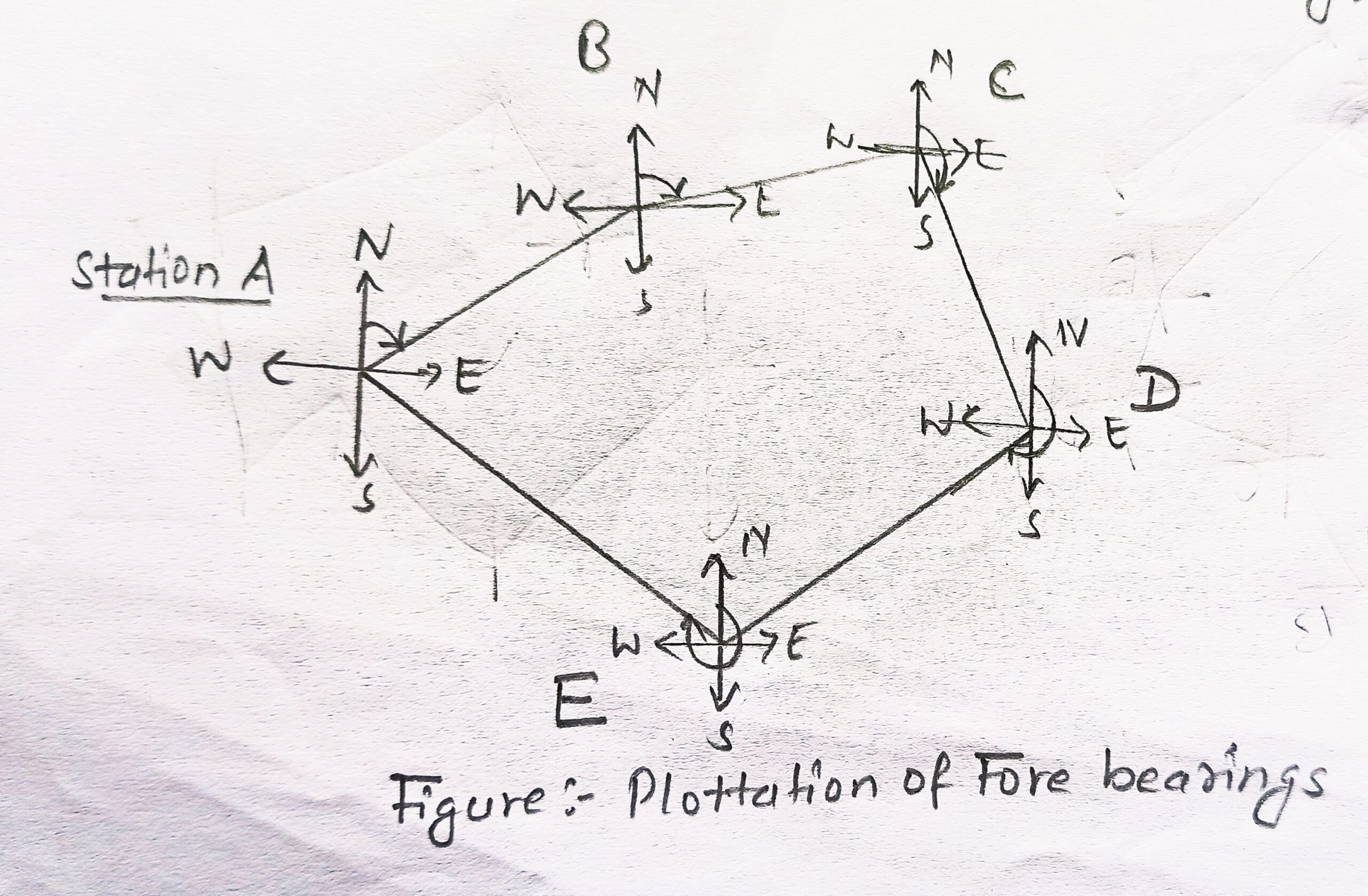### 1.7. How can we adjust angular and bearing errors?

In open traverse, adjustment of angular and bearing errors is not quite correct. But in close Traverse, we can adjust the errors accurately using the following methods:

a. Graphical Method

b. Bowditch’s Method

c. Transit Method

d. Axis Method(Analytical Method)

### 1.8. Steps for determining correct bearing and true bearing of the given closed traverse

Step 1 : Plot the traverse with the help of scale and protractor. ( Plot traversing with only fore bearings and then locate back bearings)

Step 2 : Detection of local attraction:

The presence of local attraction at any station may be detected by observing the fore bearing and back bearing of the line. If the difference between fore bearing and back bearing is 180, then both end stations are free from the local attraction. If not, the discrepancy may be due to

• An error in observation of either fore bearing or back bearing or both.

• Presence of local attraction at either station.

• Presence of local attractions at both stations.

Step 3 : Correct the local attraction and determine the correct bearing by using the analytical method:

The following steps may be followed for locating local attraction and its correction:

• Calculate the internal angle at each station from the observed bearings (in case of the closed traverse) and also correct the internal angles.

Error =|( Calculated total internal angle – Actual sum of internal angle)|

Correction = Error / n

Standard Total Internal Angle = (2n – 4) * 90

(  where n = number of incorrect internal angles)

• Observe the line whose fore and back bearing differ exactly by 180 ̊.

• Accept the bearings taken from the end stations of the line having no error, as correct.

• Calculate the back or fore bearing of the next line using the correct bearing and correct internal angles.

( when no survey line has a correct bearing, then choose the survey line having the least error and calculate correct fore bearing by adding or subtracting half of the error (least differ by 180°) to an incorrect fore bearing).

Step 4: Calculate the true bearing

True Bearing = Magnetic Bearing ± Magnetic Declination

Using +ve sign if eastern declination and –ve sign if western declination.

### 1.9. Numericals of Closed Traverse & Its Correction

Q1. The following bearings were obtained on a compass traverse.

 Line F.B (Fore bearing) B.B (Back bearing AB 51° 30′ 230° 00′ BC 162° 40′ 342° 00′ CD 104° 15′ 284° 55′ DE 165° 15′ 345° 15′ EA 251° 30′ 79° 30′

Find which stations are affected by local attraction and work out correct bearings of the lines of a closed traverse ABCDEA.

Solution,

Step 1 : Plot the given traverse bearings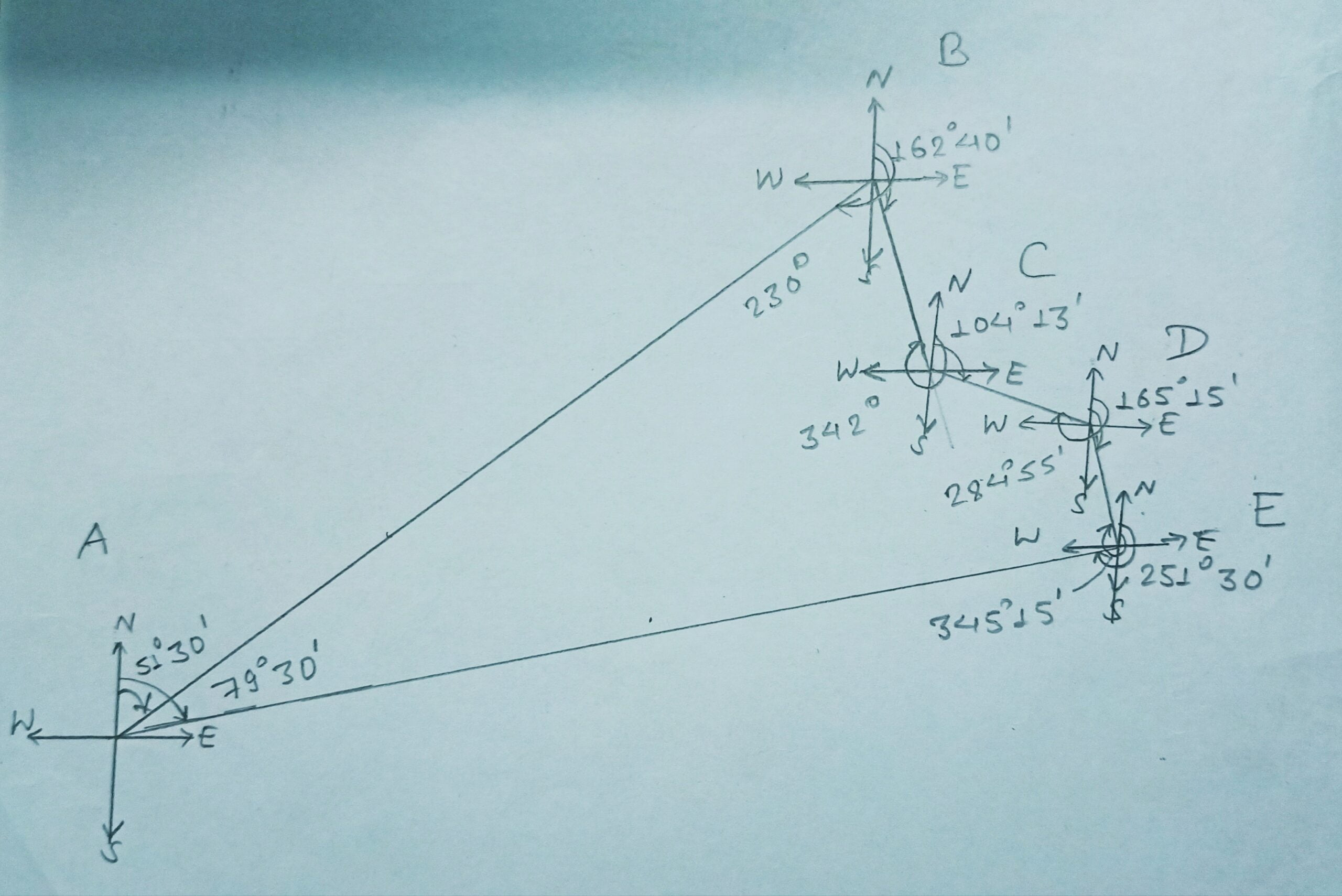Step 2: Detect the local attraction

 Line Difference ( F.B – B.B) AB 178° 30′ BC 179° 20′ CD 180° 40′ DE 180° 00′ ( Free from L.A) EF 172° 00′

Here DE survey line has a 180° difference. It simply means DE is free from Local attractions. Thus, we have to correct the remaining AB, BC, CD, & EA survey lines.

Step 3 : Calculation of internal angles, error and correction

Internal Angle at A = 79° 30′ – 51° 30′ = 28° 0′

Internal Angle at B = 230° – 162° 40′ = 67° 20′

Internal Angle at C = 342° – 104° 15′ = 237° 45′

Internal Angle at D = 284° 55′ – 165° 15′ = 119° 40′

Internal Angle at E = 345° 15′ – 251° 30′ = 93° 45′

Sum of calculated Internal Angle = 546° 30 ‘

Sum of actual Internal Angle = (2n – 4) × 90° = 540°

Error = 546° 30′ – 540° = + 6° 30′

Correction = 6° 30′ / 5 = – 1° 18′

Correct angle at A = 280° 0′ – 1° 18′ = 26° 42′

Correct angle at B = 67° 20′ – 1° 18′ = 66° 2′

Correct angle at C = 237° 45′ – 1° 18′ = 236° 27′

Correct angle at D = 119° 40′ – 1° 18′ = 118° 22′

Correct angle at E = 93° 45′ – 1° 18′ = 92° 27′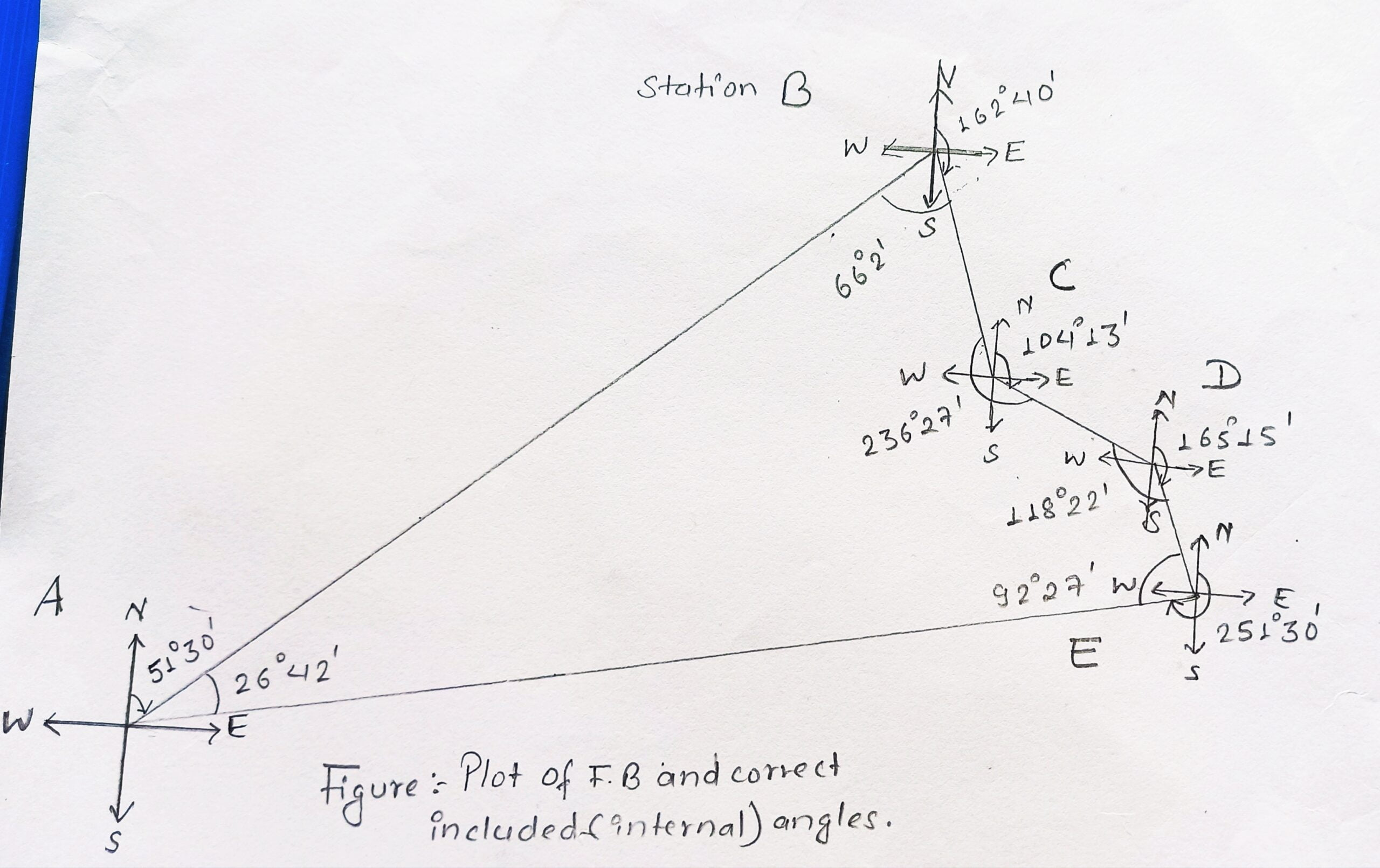Step 4 : Calculation of correct bearings

Correct bearing of survey line DE = 165° 15′

Correct bearing of survey line ED = 165° 15′ + 180° = 345° 15′

Correct bearing of survey line EA = 345° 15′ – 52° 27′ = 252° 48′

Correct bearing of survey line AE = 252° 28′ – 180° = 72° 48′

Correct bearing of survey line AB = 72° 48′ – 26° 42′ = 46° 6′

Correct bearing of survey line BA = 46° 6′ + 180° = 226° 6′

Correct bearing of survey line BC = 226° 6′ – 66° 2′ = 160° 4′

Correct bearing of survey line CB = 180° + 160° 4′ = 340° 4′

Correct bearing of survey line CD = 340° 4′ – 236° 27′ = 103° 37′

Correct bearing of survey line DC = 103° 37′ + 180° = 283° 37′

Correct bearing of survey line DE = 283° 37′ – 118° 22′ = 165° 15′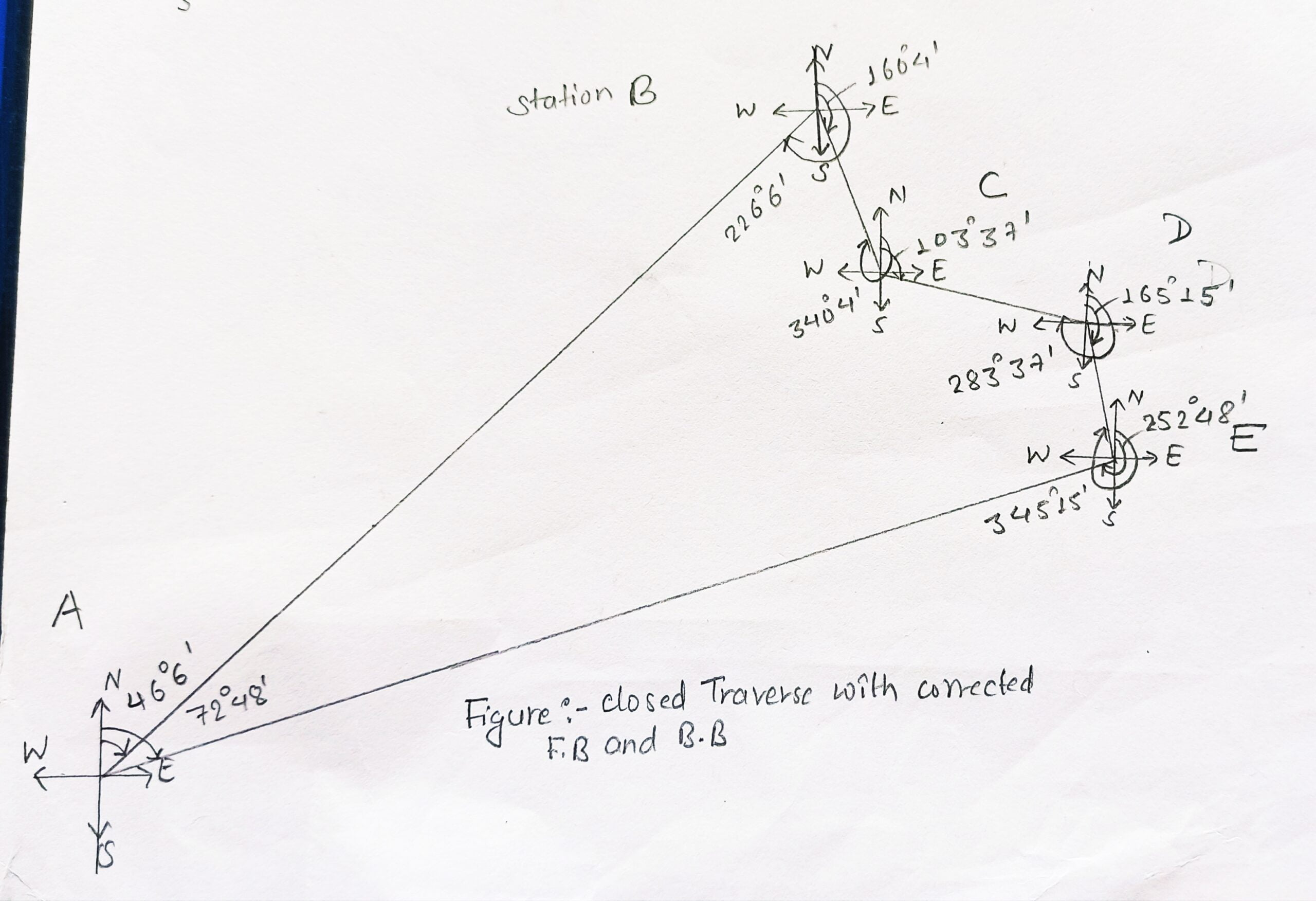Q2. The following bearings were obtained on a compass traverse.

 Line F.B B.B AB 80° 10′ 259° 00′ BC 120° 10′ 301° 50′ CD 170° 50′ 351° 50′ DE 230° 10′ 50° 10′ EA 310° 20′ 130° 20′

Find which stations are affected by local attraction and work out correct bearings of the lines of a closed traverse ABCDEA.

Solution,

Step 1 : Plot the given traverse bearings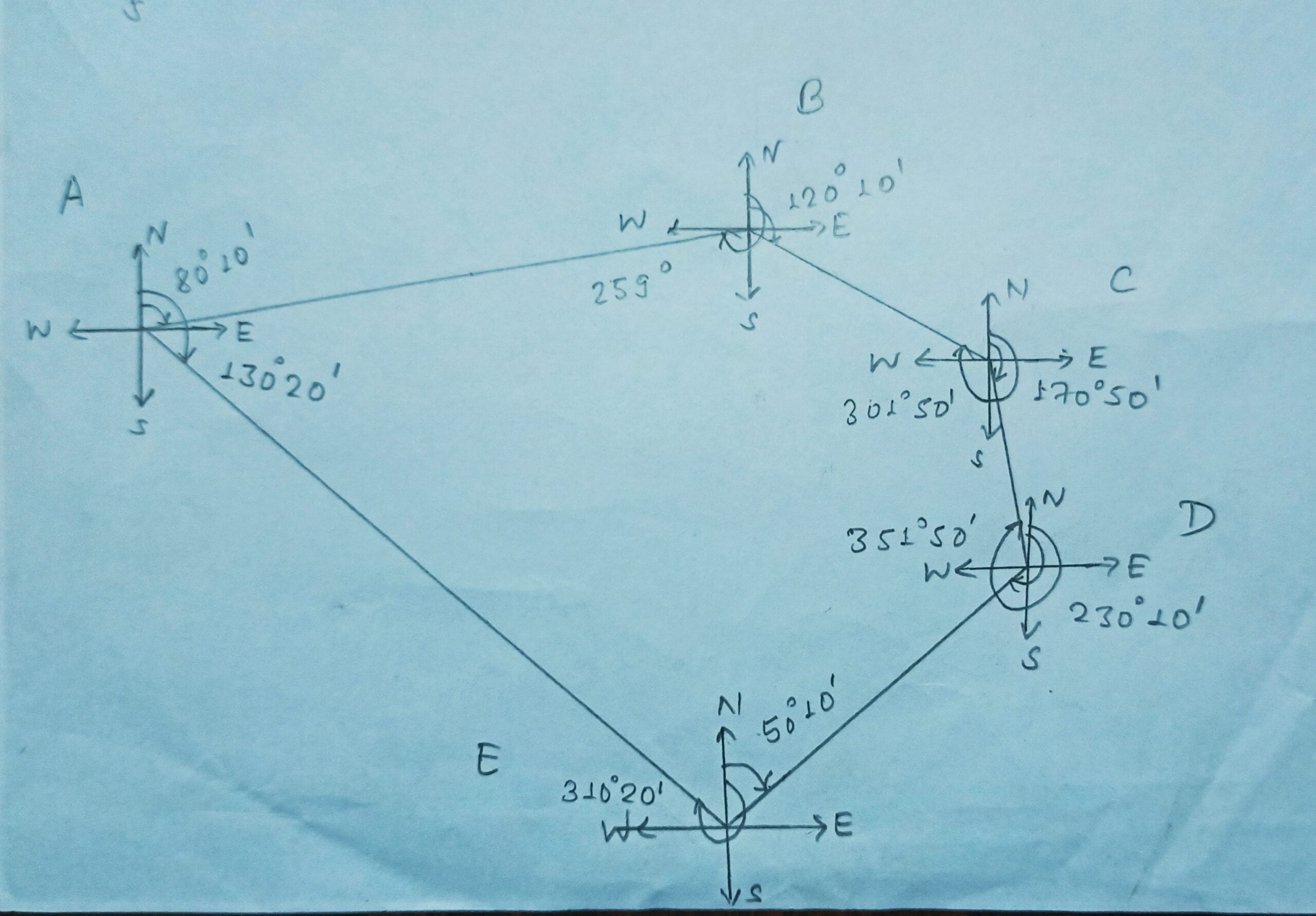Step 2: Detect the local attraction

 Line Difference ( F.B – B.B) AB 178° 50′ BC 181° 40′ CD 181° DE 180° EA 180°

As survey line, DE and EA have a 180° difference. They are free from the local attraction. Now, we have to find the correct angle and bearings of survey lines AB, BC, and CD.

Step 3 : Calculation of internal angles, error and correction

Internal Angle at A = 130° – 80° 10′ = 50° 10′

Internal Angle at B = 259° 50′ – 120° 10′ = 138° 50′

Internal Angle at C = 301° 50′ – 170° 50′ = 131° 0′

Internal Angle at D = 351° 50′ – 230° 10′ = 121° 40′

Internal Angle at E = (360° – 310° 20′) + 50° 10′ = 99° 50′

Sum of all internal angle = 541° 30′

Sum of actual Internal Angle = (2n – 4) × 90° = 540°

Error = 541° 30′ – 540° = + 1° 30′

Correction = 1° 30′ / 4 = – 0° 22′ 30″

( Because angle formed between line DE and EA is free from LA)

Correct Angle at A = 50° 10′ – 0° 22′ 30″ = 49° 47′ 30″

Correct Angle at  B = 138° 50′ – 0° 22′ 30″ = 138° 27′ 30″

Correct Angle at  C = 131° – 0° 22′ 30″ = 130° 37′ 30″

Correct Angle at  D = 121° 40′ – 0° 22′ 30″ = 121° 17′ 30″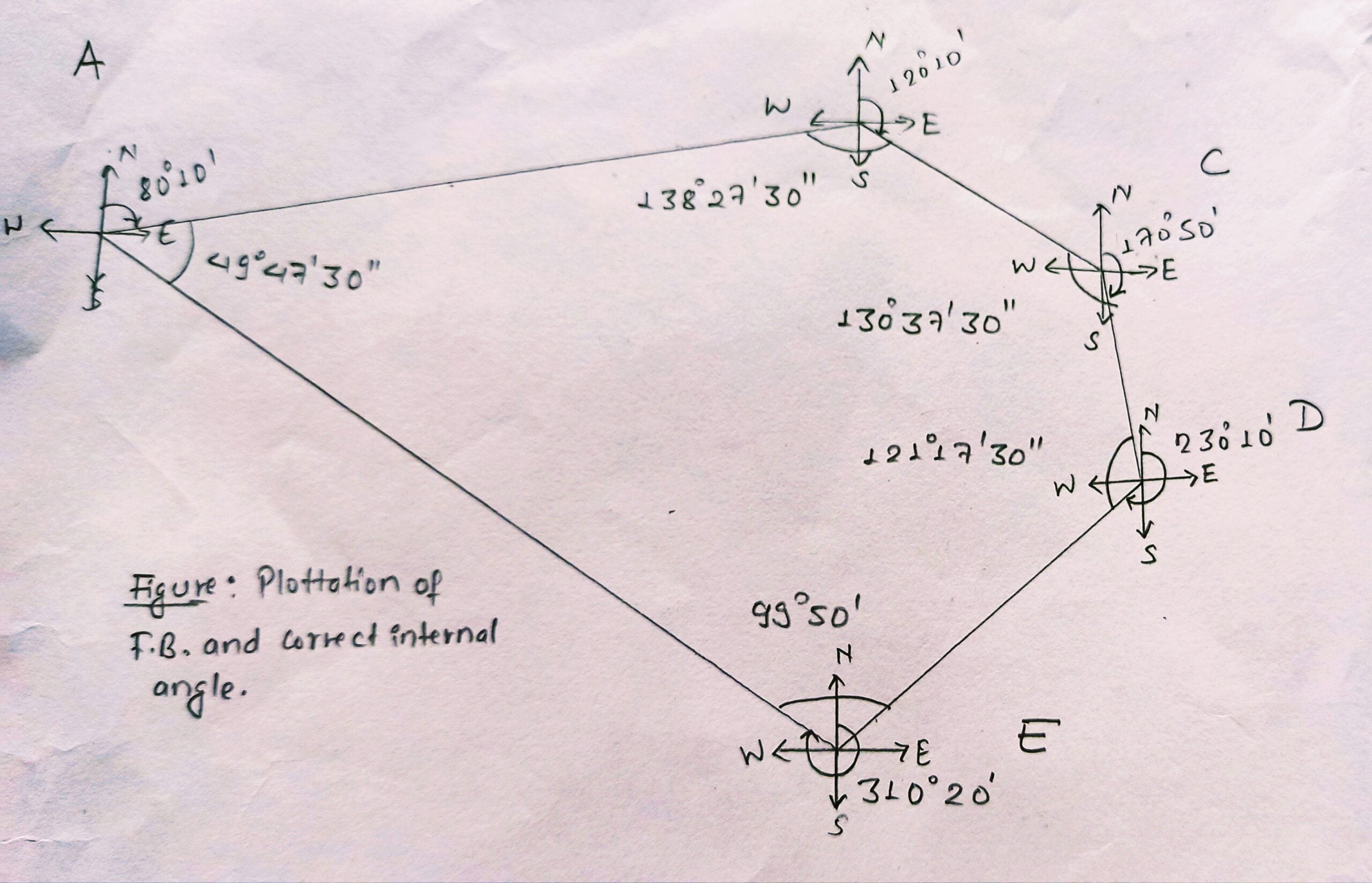Step 4 : Calculation of correct bearings

Correct Bearing of survey line DE = 230° 10′

Correct Bearing of survey line ED = 230° 10′ – 180° = 50° 10′

Correct Bearing of survey line EA = 360° – ( 99° 50′ – 50° 10′) = 310° 20′

Correct Bearing of survey line AE = 310° 20′ – 180° = 130° 20 ‘

Correct Bearing of survey line AB = 130° 20′ – 49° 47′ 30″ = 80° 32′ 30″

Correct Bearing of survey line BA = 180° + 80° 32′ 30 ” = 260° 32′ 30″

Correct Bearing of survey line BC = 260° 32′ 30″ – 138° 27′ 30″ = 122° 5′ 0″

Correct Bearing of survey line CB = 122° 5′ + 180° = 302° 5′

Correct Bearing of survey line CD = 302° 5′ – 130° 37′ 30″ = 171° 27′ 30″

Correct Bearing of survey line DC = 180° + 171° 27′ 30″ = 351° 27′ 30″

Correct Bearing of survey line DE = 351° 27′ 30″ – 121° 17′ 30″ = 230° 10’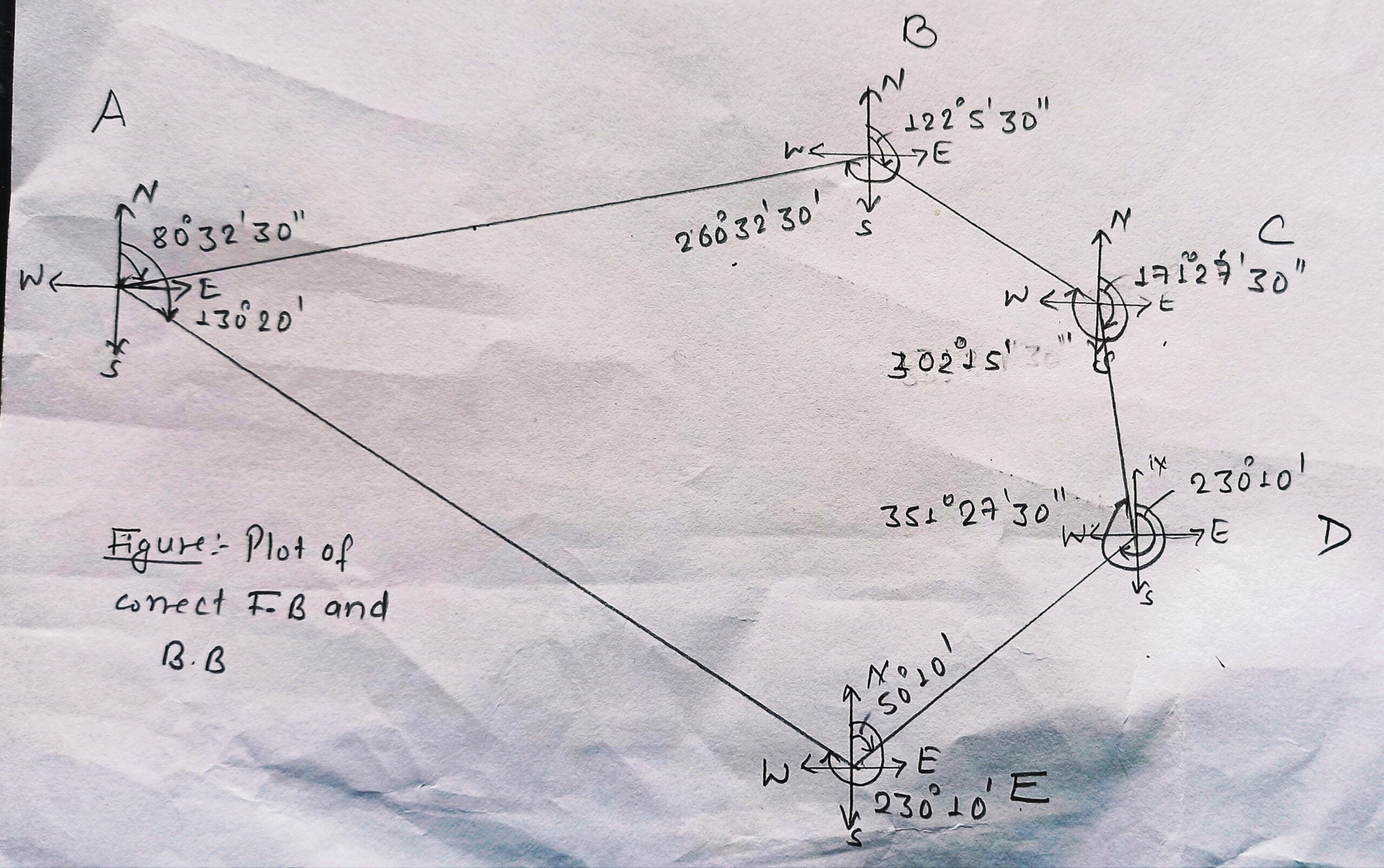Q3. The following bearings were obtained on a compass traverse.

 Line Fore bearing Back bearing AB 191° 30′ 13° 00′ BC 69° 30′ 246° 30′ CD 32° 15′ 210° 30′ DE 262° 45′ 80° 45′ EA 230° 15′ 53° 00′

Find which stations are affected by local attraction and work out correct bearings of the lines of a closed traverse ABCDEA.

Solution,

Step 1 : Plot the given traverse bearings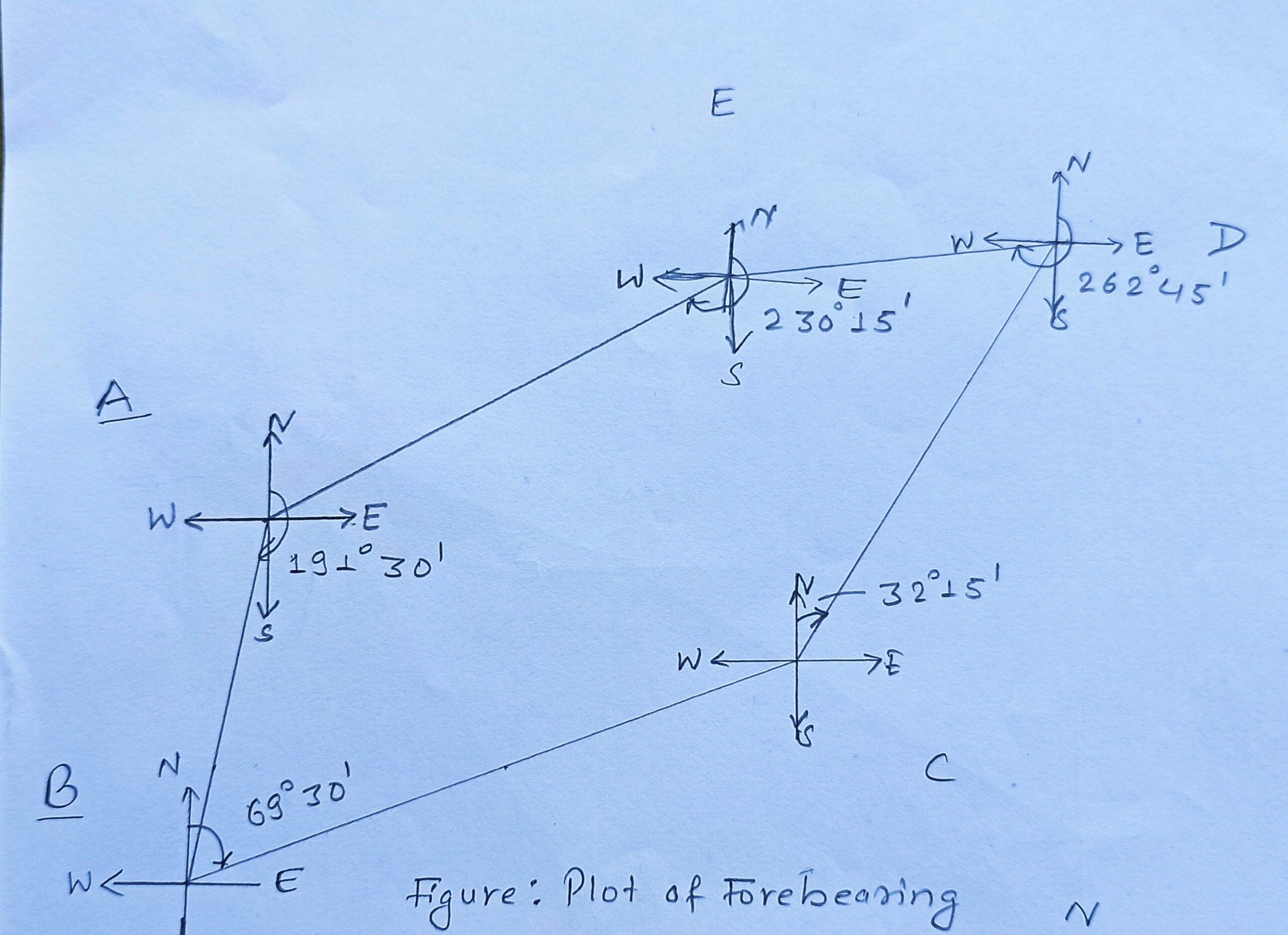Step 2: Detect the local attraction

 Line Difference ( F.B – B.B) AB 178° 30′ BC 177° CD 178° 15′ DE 182° 0′ EA 177° 15′

As no survey line has a 180° difference. They all have a local attraction. Now, we have to find the correct angle and bearings of survey lines AB, BC, CD, DE & EA.

Step 3 : Calculation of internal angles, error and correction

Internal Angle at A = 191° 30′ – 53° = 138° 30′

B = 69° 30′ – 13° = 56° 30′

C = 360° – 246° 30′ + 32° 15′ = 145° 45′

D = 262° 45′ – 210° 30′ = 52° 15′

E = 230° 15′ – 80° 45° = 149° 30′

Sum of all internal angle = 542° 30′

Sum of actual Internal Angle = (2n – 4) × 90° = 540°

Error = 542° 30′ – 540° = + 2° 30′

Correction = 2° 30′ / 5 = – 0° 30′

Correct Angle at A = 138° 30′ – 0° 30′ = 138°

Correct Angle at  B = 56° 30′ – 0° 30′ = 56°

Correct Angle at  C = 145° 45′ – 0° 30′ = 145° 15′

Correct Angle at  D = 52° 15′ – 0° 30′ = 51° 45′

Correct Angle at E = 149° 30′ – 0° 30′ = 149°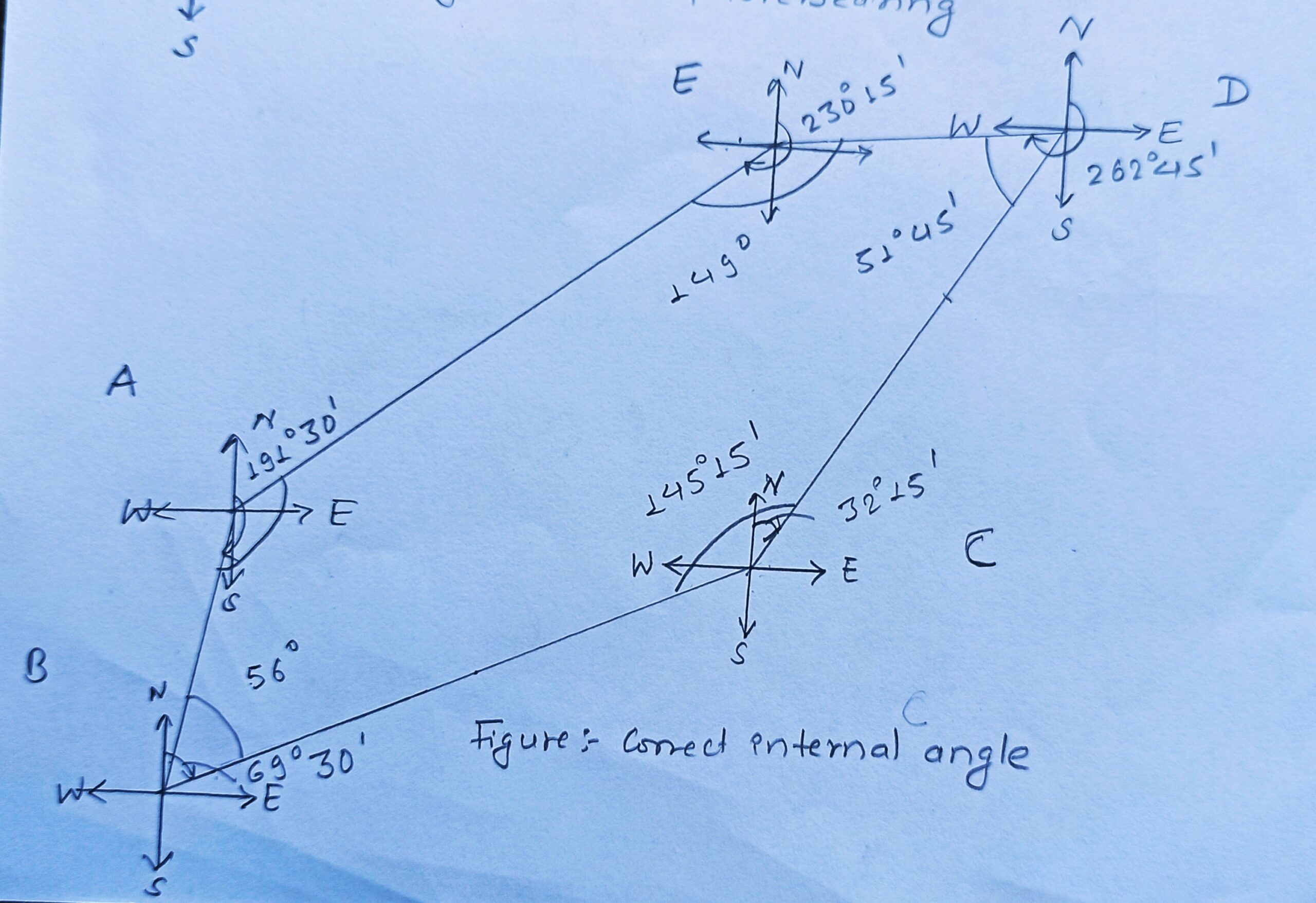Step 4 : Calculation of correct bearings

Since the difference between fore bearing and back bearing of survey line of AB least differ to 180°.

Correct bearing of survey line AB = 191° 30′ + (180° – 178° 30′)/2 = 192° 15′

Correct bearing of survey line BA = 192° 15′ – 180° = 12° 15′

Correct bearing of survey line BC = 12° 15′ + 56° = 68° 15′

Correct bearing of survey line CB = 68° 15′ + 180° = 248° 15′

Correct bearing of survey line CD = 248° 15′ + 145° 15′ – 360° = 33° 30′

Correct bearing of survey line DC = 33° 30′ + 180° = 213° 30′

Correct bearing of survey line DE = 213° 30′ + 51° 45′ = 265° 15′

Correct bearing of survey line ED = 265° 15′ – 180° = 85° 15′

Correct bearing of survey line EA = 149° + 85° 15′ = 234° 15′

Correct bearing of survey line AE = 234° 15′ -180° = 54° 15′

Correct bearing of survey line AB = 138° + 54° 15′ = 192° 15′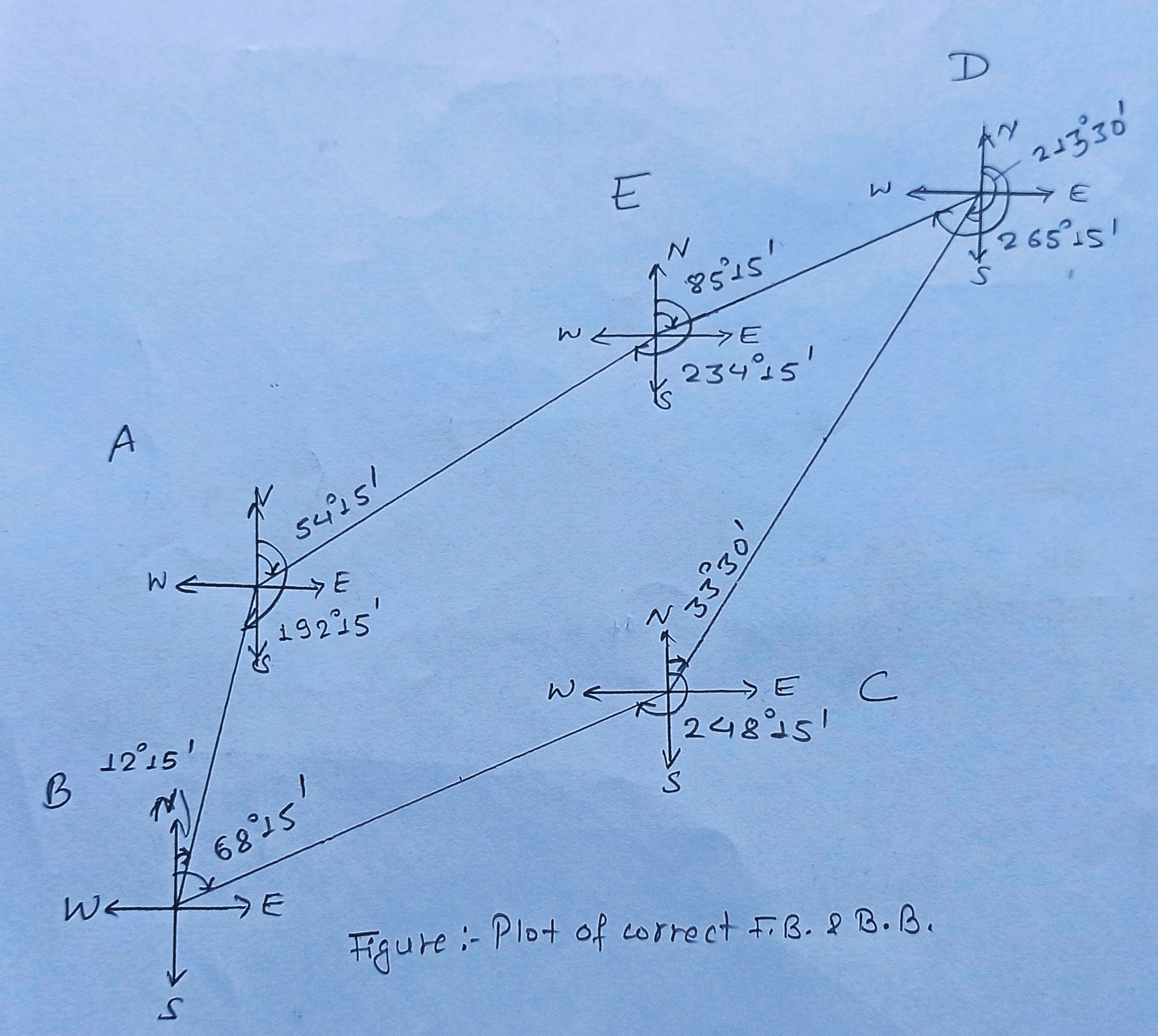He is a founder and lead author of Dream Civil International and his civil engineering research articles has been taken as source by world's top news and educational sites like USA Today, Time, The richest, Wikipedia, etc.
Latest Articles

Related Articles